• Call Now

1800-102-2727•

# Angular momentum, definition and mathematical representation, angular momentum for system of particles and rigid body, practice problems, FAQs

Why does the Earth keep on spinning? What started it spinning to begin with? And how does an ice skater manage to spin faster and faster simply by pulling her arms in? Why does she not have to exert a torque to spin faster? Questions like these have answers based on angular momentum.

Do you know why the earth keeps on spinning for thousands of years? And how the ice skater increases and decreases its rotational speed without applying any external torque. She can manage to do this only by her hands. This all can be explained by the angular momentum. Let's see what angular momentum is !

• Angular momentum
• Angular momentum in component form
• Angular Momentum of a System of Particles
• Angular Momentum of a Rigid Body Angular Momentum of Rigid Body
• Practice problems
• FAQs

## Angular momentum

Angular momentum is the rotational analogue of linear momentum. It is also known as the moment of linear momentum.Let us consider a particle is moving in x-y plane and r is the position vector of that particle at a particular point. It is moving with a translational velocity and the velocity is making an angle 0 with the position vector.

Thus, the angular momentum (moment of linear momentum) of this particle about point O is given as follows:

$\stackrel{\to }{{L}_{o}}=\stackrel{\to }{r}×\stackrel{\to }{p}$

Since $\stackrel{\to }{p}=m\stackrel{\to }{v}$

$\stackrel{\to }{{L}_{o}}=m\left(\stackrel{\to }{r}×\stackrel{\to }{v}\right)$

Using the right-hand thumb rule for the cross product, we get the direction of the angular momentum as going upwards in the positive z-direction.

We can calculate the angular momentum in two ways, either by taking the perpendicular component of the velocity vector or by taking the perpendicular component of the position vector.

On taking the perpendicular component of the velocity vector, we get the following:$|\stackrel{\to }{{L}_{o}}|=m|\stackrel{\to }{r|}|\stackrel{\to }{v}|sin\theta$

$|\stackrel{\to }{{L}_{o}}|=m|\stackrel{\to }{r|}|\stackrel{\to }{{v}_{\perp }}|$

$|\stackrel{\to }{{L}_{o}}|=|\stackrel{\to }{r|}|\stackrel{\to }{{p}_{\perp }}|$

On taking the perpendicular component of the position vector, we get the following:$|\stackrel{\to }{{L}_{o}}|=m|\stackrel{\to }{v|}|\stackrel{\to }{r}|sin\theta$

$|\stackrel{\to }{{L}_{o}}|=m|\stackrel{\to }{v|}|\stackrel{\to }{{r}_{\perp }}|$

$|\stackrel{\to }{{L}_{o}}|=|\stackrel{\to }{p|}|\stackrel{\to }{{r}_{\perp }}|$

## Angular Momentum in Component Form

$\stackrel{\to }{L}=\stackrel{\to }{r}×\stackrel{\to }{p}$

$\stackrel{\to }{r}={r}_{x}\stackrel{^}{i}+{r}_{y}\stackrel{^}{j}+{r}_{z}\stackrel{^}{k}$

$\stackrel{\to }{p}={p}_{x}\stackrel{^}{i}+{p}_{y}\stackrel{^}{j}+{p}_{z}\stackrel{^}{k}$

$\stackrel{\to }{L}=\stackrel{\to }{r}×\stackrel{\to }{p}=\left({r}_{y}{p}_{z}-{r}_{z}{p}_{y}\right)\stackrel{^}{i}+\left({r}_{z}{p}_{x}-{r}_{x}{p}_{z}\right)\stackrel{^}{j}+\left({r}_{x}{p}_{y}-{r}_{y}{p}_{x}\right)\stackrel{^}{k}$

$\stackrel{\to }{L}=\stackrel{\to }{{L}_{x}\stackrel{^}{i}}+\stackrel{\to }{{L}_{y}\stackrel{^}{j}}+\stackrel{\to }{{L}_{z}\stackrel{^}{k}}$

## Angular momentum of particle in circular path

Consider a particle of mass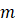moving in a circle of radius r at an angular speed ω about the z-axis in a plane parallel to the x-y plane passing through the origin O.The velocity of the particle is given by

The vector from the centre of the circle (the point O ) to the object is given by

The angular momentum about the centre of the circle is the vector product

${\stackrel{\to }{L}}_{o}={\stackrel{\to }{r}}_{O}×\stackrel{\to }{p}={\stackrel{\to }{r}}_{O}×m\stackrel{\to }{v}=rmv\stackrel{^}{k}=rmr\omega \stackrel{^}{k}=m{r}^{2}\omega \stackrel{^}{k}$

The magnitude is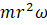and the direction is in the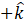-direction. Angular Momentum of a System of Particles Let us consider a system of particles with masses m1, m2, m3,.....mn having position vectors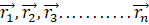, respectively, and rotating about the axis of rotation with velocities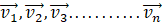, respectively.The net angular momentum of this system of particles will be the vector sum of all the angular momentum of all the individual particles.

$\stackrel{\to }{L}=\stackrel{\to }{{L}_{1}}+\stackrel{\to }{{L}_{2}}+\stackrel{\to }{{L}_{3}}+..........+\stackrel{\to }{{L}_{n}}$

$\stackrel{\to }{L}={m}_{1}\left(\stackrel{\to }{{r}_{1}}×\stackrel{\to }{{v}_{1}}\right)+{m}_{2}\left(\stackrel{\to }{{r}_{2}}×\stackrel{\to }{{v}_{2}}\right)+{m}_{3}\left(\stackrel{\to }{{r}_{3}}×\stackrel{\to }{{v}_{3}}\right)..........+{m}_{n}\left(\stackrel{\to }{{r}_{n}}×\stackrel{\to }{{v}_{n}}\right)$

## Angular Momentum of a Rigid Body Angular Momentum of Rigid Body

The angular momentum of a rigid body rotating about a fixed axis can be viewed as many small particles moving in a circular motion about the axis with different velocities. All the particles have the same angular velocity as they are a part of the same body.

A rigid body rotating about the axis can be considered as the combination of different particles having the circular motion about the same axis of rotation. So the angular momentum of the body is equal to the sum of the angular momentum of all particles present in the body. As they are on same body to angular velocity will be the same for all particles.Let us consider the particles with masses m1, m2, m3,.....mn, having position vectors, respectively, and rotating about the axis of rotation AB with velocities, respectively.

The angular momentum of the system of particles about the axis AB is given as follows:

${\stackrel{\to }{L}}_{AB}=\stackrel{\to }{{L}_{1}}+\stackrel{\to }{{L}_{2}}+\stackrel{\to }{{L}_{3}}+..........+\stackrel{\to }{{L}_{n}}$

${\stackrel{\to }{L}}_{AB}={m}_{1}\left(\stackrel{\to }{{r}_{1}}×\stackrel{\to }{{v}_{1}}\right)+{m}_{2}\left(\stackrel{\to }{{r}_{2}}×\stackrel{\to }{{v}_{2}}\right)+{m}_{3}\left(\stackrel{\to }{{r}_{3}}×\stackrel{\to }{{v}_{3}}\right)..........+{m}_{n}\left(\stackrel{\to }{{r}_{n}}×\stackrel{\to }{{v}_{n}}\right)$

For all particles,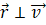${L}_{AB}={m}_{1}{r}_{1}{v}_{1}+{m}_{2}{r}_{2}{v}_{2}+{m}_{3}{r}_{3}{v}_{3}.............+{m}_{n}{r}_{n}{v}_{n}$

Since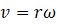${L}_{AB}={m}_{1}\omega {r}_{1}^{2}+{m}_{2}\omega {r}_{2}^{2}+{m}_{3}\omega {r}_{3}^{2}.............+{m}_{n}\omega {r}_{n}^{2}$

${L}_{AB}=\omega \sum _{i=1}^{n}{m}_{i}{r}_{i}^{2}$

Since $I=\sum _{i=1}^{n}{m}_{i}{r}_{i}^{2}$

${L}_{AB}=I\omega$

The angular momentum of a rigid body about a particular axis of rotation is the moment of inertia of that rigid body about that axis multiplied by the angular velocity of that rigid body.

The angular momentum is similar to its linear counterpart in terms of the following formula:

$\stackrel{\to }{L}=I\stackrel{\to }{\omega }$

$\stackrel{\to }{p}=m\stackrel{\to }{v}$

Where the moment of inertia is the rotational equivalent of the mass and the angular velocity is the rotational equivalent of the linear velocity.

## Practice problems

Q. Two particles A and B, each of mass m, are attached rigidly to the ends of a light rod of length l. The rod is clamped at the centre such that the system rotates about the perpendicular bisector of the rod at an angular speed 𝜔. Calculate the angular momentum of the individual particles and of the system about the axis of rotation.A.

As the axis of rotation is fixed, the particles are performing pure rotational motions.

The angular momentum of particle A is:

${L}_{A}=mvr=m\left(\omega \frac{l}{2}\right)\left(\frac{l}{2}\right)=\frac{m\omega {l}^{2}}{4}$

As the rotation is clockwise, the orientation of the angular momentum is also clockwise.

Similarly, the angular momentum of particle B is:

${L}_{B}=mvr=m\left(\omega \frac{l}{2}\right)\left(\frac{l}{2}\right)=\frac{m\omega {l}^{2}}{4}$

Angular momentum of the system,

${L}_{sys}={I}_{sys}\omega =\left[\frac{m\omega {l}^{2}}{4}+\frac{m\omega {l}^{2}}{4}\right]=\frac{m\omega {l}^{2}}{2}$

Q. A particle of mass m is projected on the horizontal ground with an initial velocity of u, making an angle 𝜃 with the horizontal. Find out the angular momentum of the particle about the point of projection when it is at the highest point of the path.A.

At this point, Hmax is the perpendicular distance of the line of motion from the point of projection.Velocity of the particle at this point is,

We know that, ${H}_{max}=\frac{{u}^{2}{sin}^{2}\theta }{2g}$

Thus, the angular momentum is as follows:

$L={r}_{\perp }p$

$⇒L=\left({H}_{max}\right)m\left(ucos\theta \right)$

$⇒L=\frac{{u}^{2}{sin}^{2}\theta }{2g}×m\left(ucos\theta \right)$

$⇒L=\frac{m{u}^{3}{sin}^{2}\theta cos\theta }{2g}$

As the direction of the angular momentum is along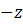axis, we can write

$⇒\stackrel{\to }{L}=\frac{m{u}^{3}{sin}^{2}\theta cos\theta }{2g}\left(-\stackrel{^}{k}\right)$

Q. Calculate the angular momentum of the Earth about its axis considering Earth as a uniform density sphere of mass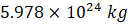and radius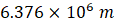A.

Time period of rotation,

Angular velocity, $\omega =\frac{2\pi }{T}=\frac{2\pi }{86400}$

Moment of inertia, $I=\frac{2}{5}M{R}^{2}$

Angular momentum,

Q. A meteor of mass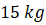enters Earth’s atmosphere and is observed by someone on the ground before it burns up in the atmosphere. The vector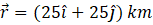gives the position of the meteor with respect to the observer. At the instant the observer sees the meteor, it has velocity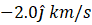, and it is accelerating at a constant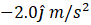along its path, which for our purposes can be taken as a straight line.What is the angular momentum of the meteor about the origin, which is at the location of the observer?

A.

The meteor is entering Earth’s atmosphere at an angle of 90.0° below the horizontal, so the components of the acceleration in the x- and y-directions are

We write the velocities using the kinematic equations.

At the instant observer sees the meteor,

Position of meteor, $\stackrel{\to }{r}=\left(25×{10}^{3}\stackrel{^}{i}+25×{10}^{3}\stackrel{^}{j}\right)$

The angular momentum is

$\stackrel{\to }{L}=\stackrel{\to }{r}×\stackrel{\to }{p}$

$=\left(25×{10}^{3}\stackrel{^}{i}+25×{10}^{3}\stackrel{^}{j}\right)×\left[15.\left(-2000\stackrel{^}{j}\right)\right]$

## FAQs

Q. Write the dimensional formula for Angular momentum.
A. The dimensional formula is $\left[M{L}^{2}{T}^{-1}\right]$.

Q. When a mass is rotating in plane about a fixed point, Its angular momentum is directed along the

a. Radius Tangent to the orbit
b. Line at angle of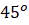to the
c. plane of rotation
d. Axis of rotation

A. When a mass is rotating in a plane about a fixed point, its angular momentum is directed along the axis of rotation.

Q. Is angular momentum a scalar quantity or a vector quantity
A. Angular momentum has both direction and magnitude and hence is a vector quantity.

Q. Suppose the angular momentum of a body is zero at about some point. Is it necessary that it will be zero at a different point?
A. No, it is not necessary that angular momentum will be zero about all the points if it is zero about any point. Assume a body travelling along the x−axis with some velocity. Now the angular momentum of the body about the origin will be zero (${r}_{\perp }=0$) It will be zero about every point lying on the x−axis. But the value of angular momentum will be non-zero about any other point having non zero y-coordinate value.

Q. For a body the angular momentum is zero about a point. Is it required that it will be zero about all the points?
A. No, it is not necessary. For example if a body is moving along y- axis the angular momentum of body will be zero about the origin as (${r}_{\perp }=0$) but about the point which is not on the y- axis the angular momentum will be non- zero.Talk to our expert
Resend OTP Timer =
By submitting up, I agree to receive all the Whatsapp communication on my registered number and Aakash terms and conditions and privacy policy